### Classical Mechanics

In Classical Mechanics, you're going to learn how to predict the motion of classical systems like springs, rockets, and helicopters by thinking in terms of force and energy. But physics is more than an accounting system for forces that predicts how objects move.

At a deeper level, it is an optimistic belief that there are rules that take systems from one moment to the next, and a way of thinking that helps us find these rules.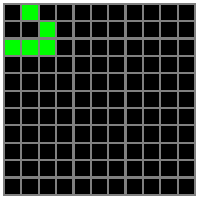A "glider" in the 2-dimensional cellular automata called Conway's Game of Life

In this quiz, we're going to look at systems called cellular automata that live and die according to simple rules on a lattice. By looking at these toy systems, we will form a clear picture of what the machinery of classical mechanics does and how it is able to describe so much of the world around us.

# Cellular Automaton

An important part of physics is deciding what information to keep track of and what to ignore. Consider, for example, air drag on a car. Billions of individual air molecules collide with a car's surface to produce a drag force, but modeling every collision would be mathematically overwhelming, even with the help of a modern computer.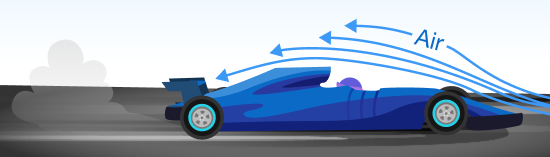The art of physics is distilling the essential qualities of a complex system into a simpler model without sacrificing predictive accuracy. Many of physics' most famous spokespersons have described this model-building process, but no one advocated for this doctrine as strongly as physicist Richard Feynman:"Others... make guesses that are very complicated, and it sort of looks as if it is all right, but I know it is not true because the truth always turns out to be simpler than you thought." 

In other words, no amount of calculation or mathematical sophistication is stand-in for developing physical intuition. In this course, we uphold Feynman's ideal as the light to guide us on our path.

# Cellular Automaton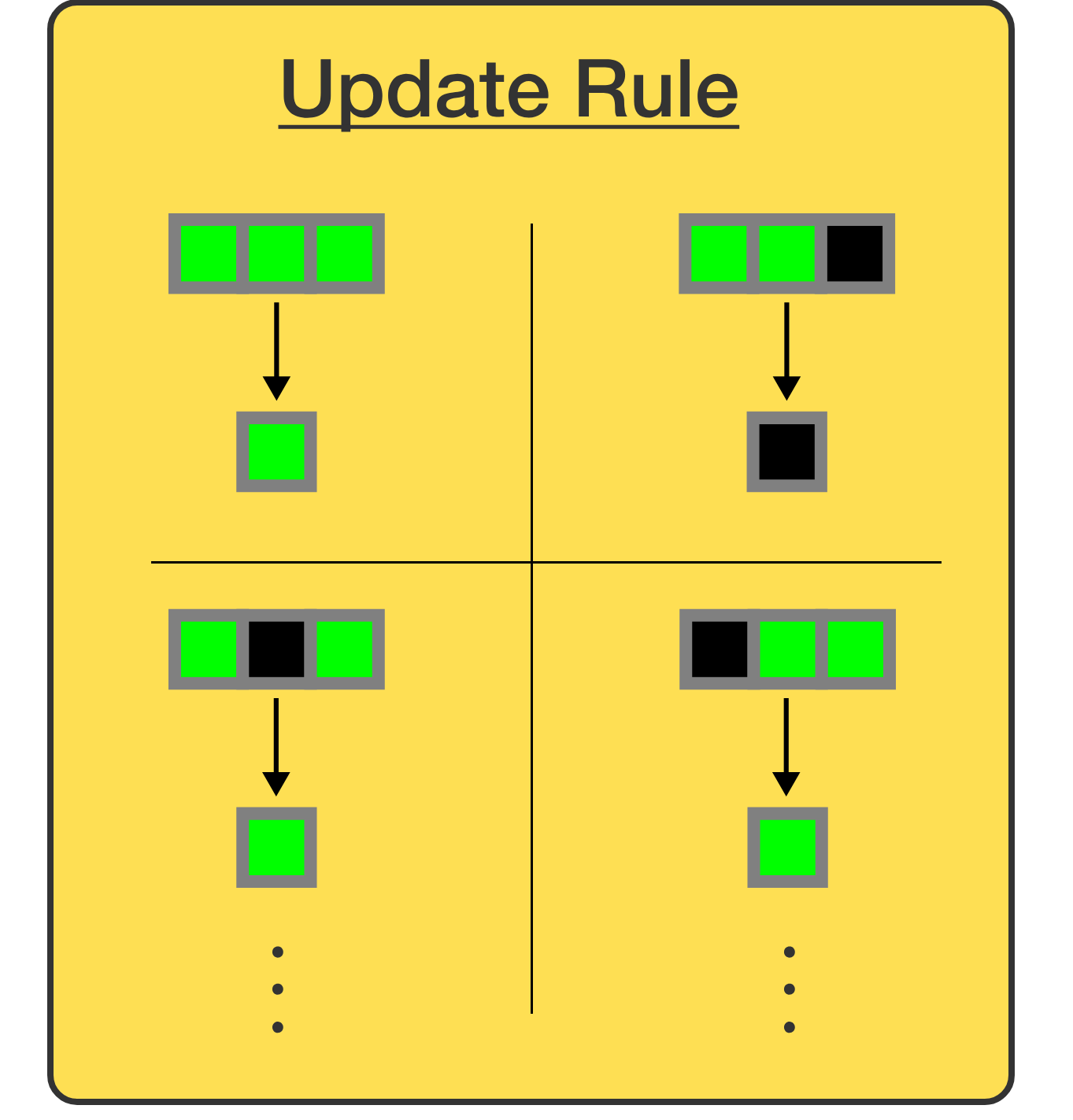Each time step, a square updates its color using the Update Rule according to the current state of its trio.

Cellular automata are a type of system that takes Feynman's doctrine to the extreme, unfolding in time according to a set of update rules that can be listed on a table. Each colored square simply looks at itself and each of its neighbors, and uses the information on the table to update its color. For simplicity, we're going to stick with $1$-dimensional automatons where each square has two neighbors.

We call each square, plus its two neighbors, a trio. If we want to define a "physics" for a cellular automaton, we need an update rule for each possible trio. For example, if a square's trio were $\{{\color{#66ff00}{\blacksquare}},{\color{#66ff00}{\blacksquare}},{\color{#333333}{\blacksquare}}\}$ it would update to $\color{#333333}{\blacksquare}$ according to the update rules in the diagram.

How many possible trios are there?

# Cellular Automaton

Once the update rule is complete with an entry for all possible trios, the dynamics of a cellular automaton are nearly unambiguous.

The last thing required is the starting color of each square. In physics, this is known as the initial state of a system. With an update rule and an initial state in hand, we have everything we need to predict any future state—the color of each square in our cellular automata.

Suppose the initial state of our system is a single green square: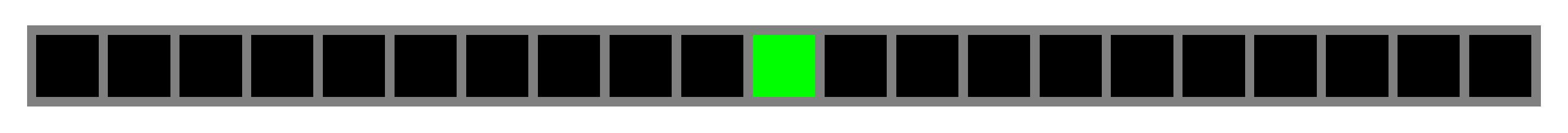What color will the green square be at the next time step using the update rule below?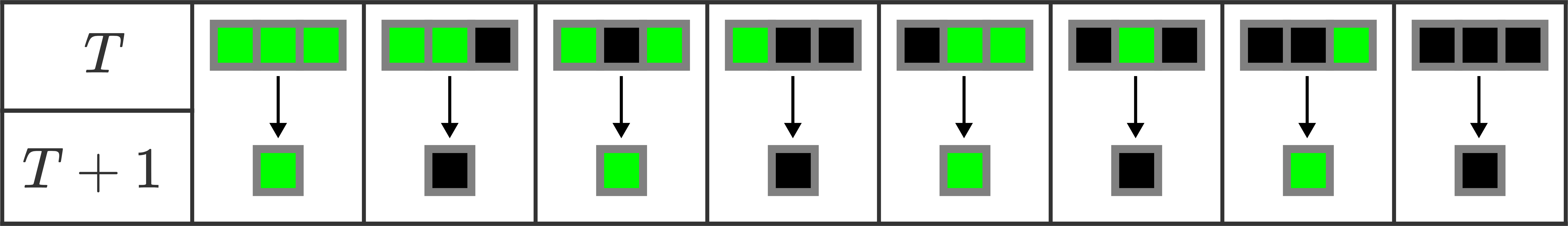# Cellular Automaton

The dynamics of a cellular automaton are fully specified by its initial state and an update rule, which encodes the state of any square one step into the future.

Nothing stops us from applying the update rule repeatedly to determine the state of the automaton at much later times. We can even string together all the states into a movie of the dynamics.

Can you figure out which of the movies below shows a cellular automaton that's following this update rule that we used in the previous question?Note: the initial state of the three cellular automata below is all squares black, except for the middle square which is green.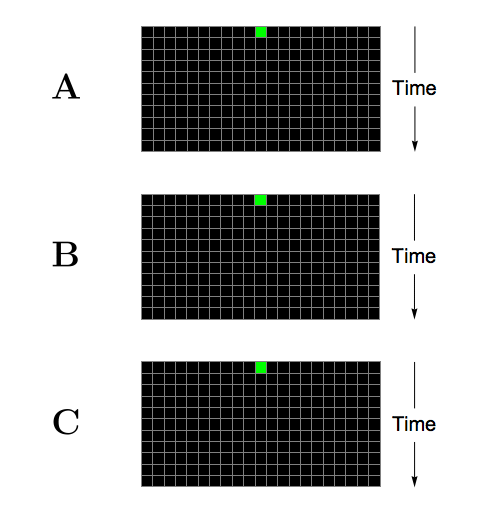# Cellular Automaton

We just saw a particular update rule that resulted in squares streaming to the left, kind of like a particle. If we wanted, we could flip that update rule in a mirror to find squares that stream to the right.

But what if we wanted an update rule that could accommodate left and right moving squares, like the system below?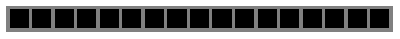For a 1-dimensional cellular automaton that updates according to the current state of its trios, there are $256$ possible update rules ($2^8$), which we can think of as $256$ possible "physics".

Is there an update rule among the $256$ that permits the rebound we see above? (try to make one!) If not, what modification would we need to make in order to see such phenomena?

# Cellular Automaton

The magic of cellular automata is that the update rule reflects all the information we know about the system, as well as any assumptions we've made about it. What's more is that the update rule encodes all the possible motions in the system.

But are there such update rules in "real" physics? Consider Newton's law of motion. It tells us that force is equal to mass times acceleration, $F = m a.$ Is this an update rule that connects two states of a system? Remember that acceleration is simply the change in velocity divided by the change in time: $a = \Delta v / \Delta t.$ Taking this into account, Newton's law becomes $\boxed{v_\text{final} = v_\text{initial} + \dfrac{F\Delta t}{m}},$ a simple update rule!

Reduced to its essence, classical mechanics is a two-fold endeavor: understanding the lessons this update rule contains about the natural world, and learning to solve it for the forces we find in nature.

There is one caveat that we glossed over. In nature it seems that time and space aren't discrete like they are in cellular automata, they're continuous. This means we have to make the timestep $\Delta t$ infinitesimally small, which changes the discrete update rule into a differential equation: $m\frac{dv}{dt} = F.$

# Cellular Automaton

Although this will be our only encounter with cellular automata in this course, they are an archetype of how complexity arises from simple update rules. For instance, consider the cellular automaton better known as the Game of Life, which has the following update rule:

1. Any green cell with exactly 2 or 3 green neighbors stays green
2. Any other green cell turns black
3. Any black cell with exactly 3 green neighbors turns green

The simplicity of the update rule is superficial. Hidden beneath is a rich universe of lattice creatures that it encodes, which contains patterns that move themselves across the lattice, patterns that spit out other patterns, and patterns that make copies of themselves.

As but one example, the contraption below generates lattice gliders at regular intervals: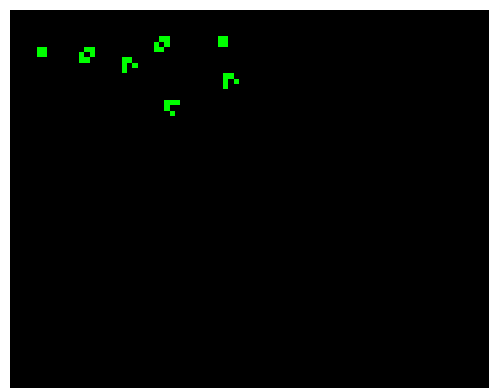Despite the simplicity of their update rules, cellular automata can give rise to complex behavior.

In the final quiz, we'll use Newton's laws, the central update rule in Classical Mechanics, to illustrate complexity encoded by simple rules—the mysterious case of two clocks talking to each other through a wall.

See you there.

×